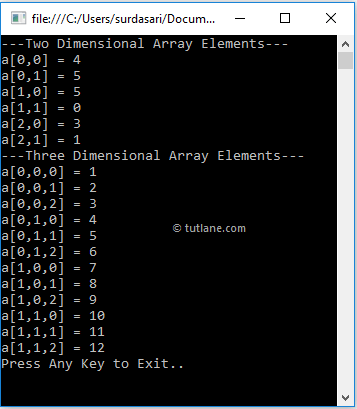# C# Multidimensional Arrays

As discussed in the previous chapter, Arrays in C# will support multi-dimensional arrays.  In c#, a multidimensional array is an array that contains more than one dimension to represent the elements in a tabular format like rows and columns.

In c#, multidimensional arrays can support either two or three-dimensional series. To create multi-dimensional arrays, we need to use a comma (,) separator inside the square brackets.

## C# Multi-Dimensional Array Declaration

In c#, Multidimensional Arrays can be declared by specifying the data type of elements followed by the square brackets [] with comma (,) separator. The following are examples of creating two or three-dimensional arrays in the c# programming language.

// Two Dimensional Array
int[,] arr = new int[4, 2];
// Three Dimensional Array
int[, ,] arr1 = new int[4, 2, 3];

If you observe the above examples, we created a two-dimensional array (arr) with 4 rows, 2 columns, and another array (arr1) with three dimensions 4, 2, 3.

## C# Multi-Dimensional Array Initialization

In c#, we can initialize arrays upon declaration. Following are the different ways of declaring and initializing multidimensional arrays in the c# programming language.

// Two Dimensional Integer Array
int[,] intarr = new int[3, 2] {
{ 4, 5 },
{ 5, 0 },
{ 3, 1 }
};
// Two Dimensional Integer Array without Dimensions
int[,] intarr1 = new int[,] { { 4, 5 }, { 5, 0 }, { 3, 1 } };
// Three Dimensional Array
int[, ,] array3D = new int[2,2,3] { { { 1, 2, 3 }, { 4, 5, 6 } },
{ { 7, 8, 9 }, { 10, 11, 12 } }
};
// Three Dimensional Array without Dimensions
int[, ,] array3D1 = new int[,,] { { { 1, 2, 3 }, { 4, 5, 6 } },
{ { 7, 8, 9 }, { 10, 11, 12 } }
};

If you observe the above examples, we declared and initialized a two-dimensional array with 3 rows and 2 columns and a three-dimensional array with 2, 2, 3 dimensions.

In the second and fourth statements, while declarations, we initialized arrays with values without specifying any dimensions. Here, the dimensions of an array can be determined by the number of elements, so the dimension initializers are not required if we assign elements during the initialization.

The following diagram will illustrate more details about how multidimensional arrays will be divided into rows and columns in the c# programming language.If you observe the above diagram, we defined a two-dimensional array with 3 rows, 2 columns and inserting the elements in an array based on the defined number of elements.

## C# Access Multidimensional Array Elements

In c#, we can access the values of multidimensional arrays by using the row index and column index values.

Following is the example of accessing elements from multidimensional arrays in c# programming language based on our requirements.

// Two Dimensional Array
int[,] arr2D = new int[3, 2] {
{ 4, 5 },
{ 5, 0 },
{ 3, 1 }
};
// Three Dimensional Array
int[, ,] array3D = new int[2,2,3] {
{ { 1, 2, 3 }, { 4, 5, 6 } },
{ { 7, 8, 9 }, { 10, 11, 12 } }
};
Console.WriteLine(arr2D[1, 0]); // 5
Console.WriteLine(array3D[1,1,1]); // 11

If you observe the above example, we are accessing elements of two or three-dimensional arrays by passing index values.

## C# Multidimensional Array Example

Following is the example of using multidimensional arrays in c# programming language to represent the elements in an array with multiple dimensions.

using System;

namespace Tutlane
{
class Program
{
static void Main(string[] args)
{
// Two Dimensional Array
int[,] array2D = new int[3, 2] { { 4, 5 }, { 5, 0 }, { 3, 1 } };
// Three Dimensional Array
int[, ,] array3D = new int[2, 2, 3] { { { 1, 2, 3 }, { 4, 5, 6 } }, { { 7, 8, 9 }, { 10, 11, 12 } } };
Console.WriteLine("---Two Dimensional Array Elements---");
for (int i = 0; i < 3; i++)
{
for (int j = 0; j < 2; j++)
{
Console.WriteLine("a[{0},{1}] = {2}", i, j, array2D[i, j]);
}
}
Console.WriteLine("---Three Dimensional Array Elements---");
for (int i = 0; i < 2; i++)
{
for (int j = 0; j < 2; j++)
{
for (int k = 0; k < 3; k++)
{
Console.WriteLine("a[{0},{1},{2}] = {3}", i, j, k, array3D[i, j, k]);
}
}
}
Console.WriteLine("Press Enter Key to Exit..");
}
}
}

If you observe the above example, we created two and three-dimensional arrays and got those array values using for loop in c#.

When we execute the above c# program, we will get the result as shown below.If you observe the above result, we are able to get the two or three-dimensional arrays by using respective index values based on our requirements.

In c#, multidimensional arrays are slower than single-dimensional arrays while accessing elements from arrays. Instead of multidimensional arrays, we can use jagged arrays in c# to improve the application's performance.

This is how we can use multidimensional arrays in our c# applications based on our requirements.